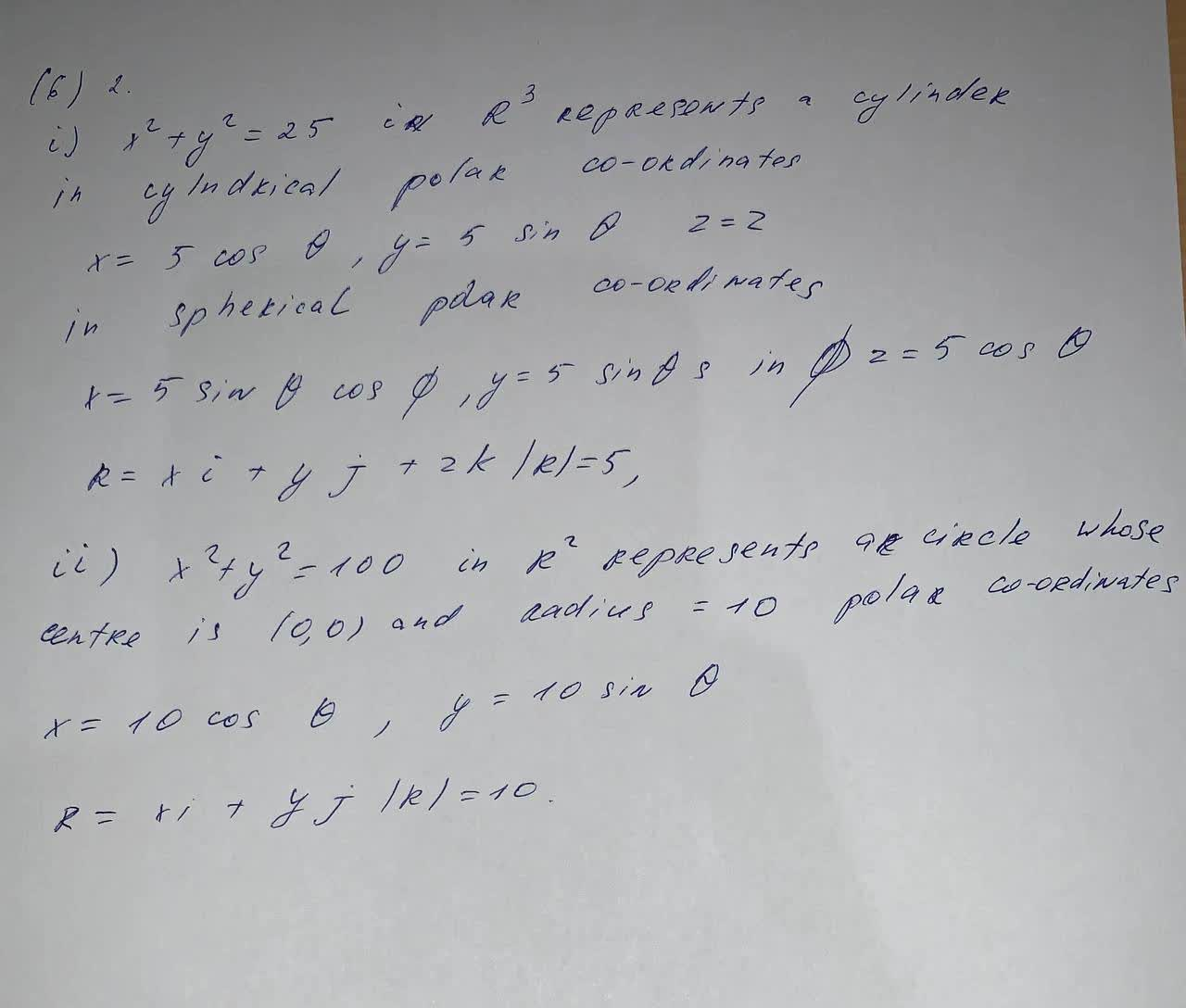Write an equation in the indicated coordinate system. Also write a parametric equation and a vector equation for the following equation x^{2}+y^{2}=100 in R^{2}, polar coordinatesBevan Mcdonald 2021-09-01 Answered
Write an equation in the indicated coordinate system. Also write a parametric equation and a vector equation for the following equation
$$\displaystyle{x}^{{{2}}}+{y}^{{{2}}}={100}\in{R}^{{{2}}}$$, polar coordinates

• Questions are typically answered in as fast as 30 minutes

Solve your problem for the price of one coffee

• Math expert for every subject
• Pay only if we can solve itLaaibah Pitt

Equation in the indicated coordinate system is written below